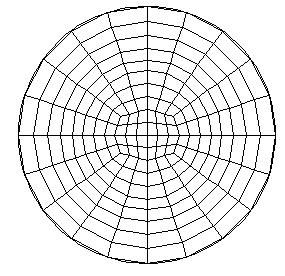# Circle

Applies to: Surfaces

Summary: Produces a circle-primitive mesh for a surface

Syntax:

Surface <range> Scheme [Sector] Circle [Interval <int>] [fraction <double>]

Discussion:

The Circle scheme is used in regions that should be meshed as a circle. A "circle" consists of a single loop of bounding curves containing an even number of intervals. Thus, the circle scheme can be applied to circles, ellipses, ovals, and regions with "corners" (e.g. polygons). The bounding curves should enclose a convex region. Non-planar bounding loops can also be meshed using the circle primitive provided the surface curvature is not too great. The mesh resembles that obtained via polar coordinates except that the cells at the "center" are quadrilaterals, not triangles. See Figure 1 for an example of a circle mesh. Radial grading of the mesh may be achieved via the optional [intervals] input parameter. The Fraction option has the range 0 < fraction < 1 and defaults to 0.5. Fraction determines the size of the inner portion of the circle mesh relative to the total radius of the circle. The sector option was added to revert to legacy behavior which is not recommended.Figure 1. Circle Primitive Mesh